# Gymathics 1: Basic GymathicsFREE

23 Lessons
4.6(52)

This course is free and editable. Yours to re-brand and tailor to your needs!

This course features basic math concepts and principles that are used for maritime-related calculations.

## Gymathics 1: Basic Gymathics Lessons

Click through the microlessons below to preview this course. Each lesson is designed to deliver engaging and effective learning to your team in only minutes.

1. Fractions: Lesson 1
2. Fractions: Lesson 2
3. Fractions: Lesson 3
4. Fractions: Lesson 4
5. Fractions: Lesson 5
6. Fractions: Lesson 6
7. Fractions: Lesson 7
8. Fractions: Lesson 8
9. Fractions: Lesson 9
10. Percent: Lesson 1
11. Percent: Lesson 2
12. Percent: Lesson 3
13. Integers: Lesson 1
14. Integers: Lesson 2
15. Exponents: Lesson 1
16. Exponents: Lesson 2
21. Algebra: Lesson 1
22. Algebra: Lesson 2
23. Algebra: Lesson 3

### Like what you see?

This course is free and completely editable. Update the text, add your own slides or re-brand the entire course — with our no-code authoring tool, the sky’s the limit!

Follow the interactions on each screen or click the arrows to navigate between lesson slides.

## Gymathics 1: Basic Gymathics course excerpts

### Fractions: Lesson 1

Gymathics 1: Basic Gymathics Course - Lesson Excerpt

Fractions: Lesson 1

What is a fraction? Represents equal parts of a whole Represents parts of a set or a collection The word "fraction" has been derived from the Latin ‘fractus’ which means “broken”.

In the fraction 4/5, which is the numerator?

In the fraction 3/7, which is the denominator?

In a class of 50 students, 27 are girls and 23 are boys. Which fraction represents the girls in the class?

Jane's score in her Math exam is 17/20. Which of the following statements is correct?

### Fractions: Lesson 2

Gymathics 1: Basic Gymathics Course - Lesson Excerpt

Fractions: Lesson 2

What type of fraction is 5/6?

Which of the following fractions is an improper fraction?

Which of the following fractions has a value that is closest to 1?

Which of the following fractions has a value that is closest to 0?

Which of the following fractions has the greatest value?

### Fractions: Lesson 3

Gymathics 1: Basic Gymathics Course - Lesson Excerpt

Fractions: Lesson 3

Example 1: Convert 5/2 to mixed number Divide 5 by 2 The answer is 2 remainder 1 2 is the whole number, 1 is the numerator Final answer: 2 1/2

Example 2: Express 9/7 in mixed form. Divide 9 by 7 The answer is 1 remainder 2 1 is the whole number, 2 is the numerator Final answer: 1 2/7

Example 1: Convert 3 1/4 to improper fraction Multiply 3 (whole number) by 4 (denominator) The product is 12. Add 1 (numerator). The sum is 13. This is the "new" numerator. Final Answer: 13/4

Example 2: Convert 7 3/5 to improper fraction Multiply 7 (whole number) by 5 (denominator) The product is 35. Add 3 (numerator). The sum is 38. This is the "new" numerator. Final Answer: 38/5

19/3 in mixed form is 6 2/3

Which of the following improper fractions is equal to 3 1/2?

Which of the following improper fractions is equal to 4 3/7?

### Fractions: Lesson 4

Gymathics 1: Basic Gymathics Course - Lesson Excerpt

Fractions: Lesson 4

The simplest form of 7/21 is 1/3

The simplest form of 18/4 is 4 2/4.

The simplest form of 12/15 is 3/5

The simplest form of 27/36 is 3/4

### Fractions: Lesson 5

Gymathics 1: Basic Gymathics Course - Lesson Excerpt

Fractions: Lesson 5

### Fractions: Lesson 6

Gymathics 1: Basic Gymathics Course - Lesson Excerpt

Fractions: Lesson 6

Example 1: What is the product of 2/3 an 1/6? Multiply the numerators (2 and 1). The product is 2. Multiply the denominators (3 and 6). The product is 18. The answer is 2/18. Next, simplify the answer. The simplified form of 2/18 is 1/9.

Example 2: What is the product of 4/7 an 2/8? Multiply the numerators (4 and 2). The product is 8. Multiply the denominators (7 and 8). The product is 56. The answer is 8/56. Next, simplify the answer. The simplified form of 8/56 is 1/7.

### Fractions: Lesson 7

Gymathics 1: Basic Gymathics Course - Lesson Excerpt

Fractions: Lesson 7

Example 1: Divide 1/3 by 2/8 Get the reciprocal of the second fraction (2/8). The answer is 8/2. Multiply the reciprocal (8/2) by the first fraction (1/3). Multiply the numerators (8 and 1). The product is 8. Multiply the denominators (2 and 3). The product is 6. Simplify the answer (8/6). Final answer is 1 1/3.

Example 2: Divide 4/7 by 1/9 Get the reciprocal of the second fraction (1/9). The answer is 9/1. Multiply the reciprocal (9/1) by the first fraction (4/7). Multiply the numerators (9 and 4). The product is 36. Multiply the denominators (1 and 7). The product is 7. Simplify the answer (36/7). Final answer is 5 1/7.

The quotient of 1/4 divided by 1/5 is 1 1/4.

The quotient of 3/8 divided by 4/9 is 1/6.

### Fractions: Lesson 8

Gymathics 1: Basic Gymathics Course - Lesson Excerpt

Fractions: Lesson 8

Example 1: Convert 5/6 to decimal form. Divide the numerator (5) by the denominator (6). The answer is 0.83

Example 2: Convert 15/30 to decimal form. Divide the numerator (15) by the denominator (30). The answer is 0.5.

Example 3: Convert 3 3/4 to decimal form. Divide the numerator (3) by the denominator (4). The answer is 0.75. Add the quotient (0.75) to the whole number (3). Final answer: 3.75

### Fractions: Lesson 9

Gymathics 1: Basic Gymathics Course - Lesson Excerpt

Fractions: Lesson 9

Example 1: What is 4/5 in percent? Divide the numerator (4) by the denominator (5). Multiply the quotient (0.8) by 100. Then add "%" symbol. Answer: 80%

Example 2: What is 7/9 in percent? Divide the numerator (7) by the denominator (9). Multiply the quotient (0.78) by 100. Then add "%" symbol. Answer: 78%

### Percent: Lesson 1

Gymathics 1: Basic Gymathics Course - Lesson Excerpt

Percent: Lesson 1

Example 1: What is 30% of 60? Convert the percentage (30%) to decimal. Multiply the decimal (0.3) to the whole (60). Final answer: 18

Example 2: What is 78% of 520? Convert the percentage (78%) to decimal. Multiply the decimal (0.78) to the whole (520). Final answer: 405.6

Example 3: What is 150% of 45? Convert the percentage (150%) to decimal. Multiply the decimal (1.5) to the whole (45). Final answer: 67.5

### Percent: Lesson 2

Gymathics 1: Basic Gymathics Course - Lesson Excerpt

Percent: Lesson 2

Example 1: In a class of 50 students, 32 are girls. What percent of the class are girls? Divide the part (32) by the whole (50). Multiply the quotient (0.64) by 100. Add the "%" symbol. Final answer: 64%

Example 2: Marcus got a score of 22 points out of a 30-item test correctly. What is his equivalent grade in percent? Divide the part (22) by the whole (30). Multiply the quotient (0.73) by 100. Add the "%" symbol. Final answer: 73%

### Percent: Lesson 3

Gymathics 1: Basic Gymathics Course - Lesson Excerpt

Percent: Lesson 3

Example 1: There are 92 women who watched the musical play. If 40% of the audience are women, how many people are in the audience? Multiply the part (92) by 100. Divide the product (9,200) by the percent (40). Final answer: 230

Example 2: Vic has watched 25 Korean movies listed on Netflix. If he has watched only 5% of the movies on Netflix, how many movies are there in total? Multiply the part (25) by 100. Divide the product (2,500) by the percent (5). Final answer: 500

### Integers: Lesson 1

Gymathics 1: Basic Gymathics Course - Lesson Excerpt

Integers: Lesson 1

Example 1: (-4) + (-5) Proceed with usual addition (4 + 5) Copy the sign of the numbers (-) Final answer: (-9)

Example 2: 9 + 11 Proceed with usual addition (9 + 11) Copy the sign of the numbers (+) Final answer: 20

Example 1: (-2) + 7 Subtract the numbers (7 - 2) Copy the sign of the greater number (+) Final answer: 5

Example 2: 5 + (-11) Subtract the numbers (11 - 5) Copy the sign of the greater number (-) Final answer: -6

Example 1: 7 - (-2) Change the sign of the second number (+2) Proceed to addition (7 + 2) Final answer: 9

Example 2: (-7) - 1 Change the sign of the second number (-1) Proceed to addition (-7 + -1) Final answer: -8

### Integers: Lesson 2

Gymathics 1: Basic Gymathics Course - Lesson Excerpt

Integers: Lesson 2

Example 1: 5 x (-5) x (-2) The number of negative factors is EVEN (2 factors). The answer is 50.

Example 2: What is the quotient of (-2) x (-2) divided by 4 x (-1) The number of negative factors is ODD (3 factors). The answer is (-1).

### Exponents: Lesson 1

Gymathics 1: Basic Gymathics Course - Lesson Excerpt

Exponents: Lesson 1

Which of the following exponents is equal to 64?

Which of the following exponents is equal to 64?

Which of the following exponents is equal to 64?

Which of the following exponents is equal to 81?

Which of the following exponents is equal to 81?

Which of the following exponents is equal to 81?

### Exponents: Lesson 2

Gymathics 1: Basic Gymathics Course - Lesson Excerpt

Exponents: Lesson 2

To convert this to a regular number, shift the decimal place 6 places to the right. The exponent tells you how many times you would shift the decimal place

To convert this to scientific notation, shift the decimal from the right to the left until it reaches the first digit. The number of shifts is the exponent.

To convert this to a regular number, shift the decimal place 3 places to the left. The exponent tells you how many times you would shift the decimal place.

To convert this to scientific notation, shift the decimal place to the left until it reaches the first non-zero digit (3). The number of shifts is the negative exponent.

Gymathics 1: Basic Gymathics Course - Lesson Excerpt

When simplifying radicals, write down all the factors of the number.

In simplifying square roots, find the numbers that appear THRICE in the factors.

Gymathics 1: Basic Gymathics Course - Lesson Excerpt

Gymathics 1: Basic Gymathics Course - Lesson Excerpt

Gymathics 1: Basic Gymathics Course - Lesson Excerpt

### Algebra: Lesson 1

Gymathics 1: Basic Gymathics Course - Lesson Excerpt

Algebra: Lesson 1

### Algebra: Lesson 2

Gymathics 1: Basic Gymathics Course - Lesson Excerpt

Algebra: Lesson 2

### Algebra: Lesson 3

Gymathics 1: Basic Gymathics Course - Lesson Excerpt

Algebra: Lesson 3

## Course media gallery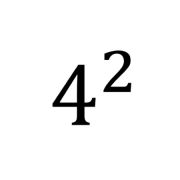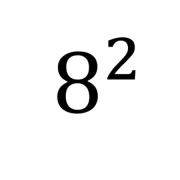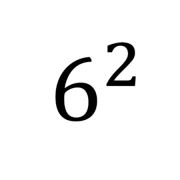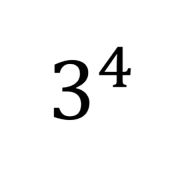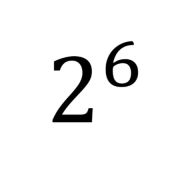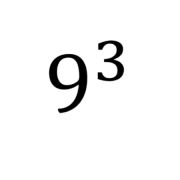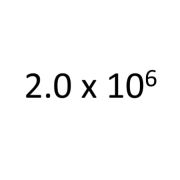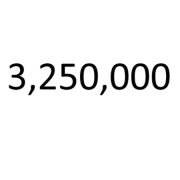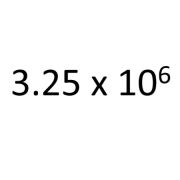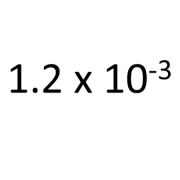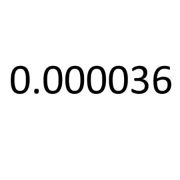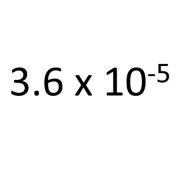##### Gymathics 1: Basic Gymathics

Course rating

Good,easy and understanding

Interesting lesson

Good

Good

## EdApp is easy to use and free for you and your team. No credit card required.

or book a demo with us today